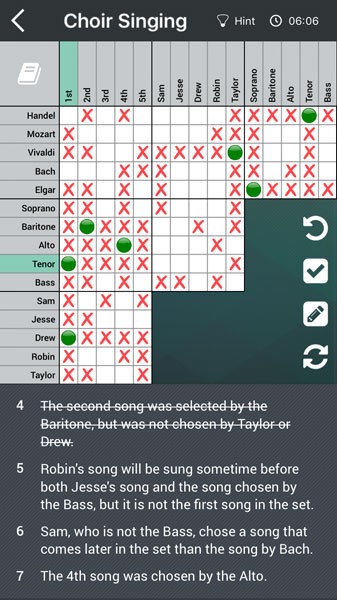# Cross Matching Answers In Logic Puzzles

In the previous posts, we’ve been talking a lot about how you can match pieces of information from one section of the grid to another. To further expand on this, we can use a thing called cross matching when it comes to true answers. In order to explain this, take a look at the grid below:We can see along the top line that Handel and Tenor are true. If we look down the left side of the grid we can also see that Tenor and 1st are true. This then leads us onto the realization that Handel and 1st must be true because the horizontal and vertical lines of true answers must intersect. If we now know that the vertical colum for 1st is Handel, Tenor, Drew – we then know that the horizontal row for Handel must have the same values . . so 1st, Drew, Tenor.

Following the fact that the true answers must intersect, we then know we can fill in Tenor and Drew as a true answer as well . .  loads of true answers filled in simply by intersecting the lines created by two truths.